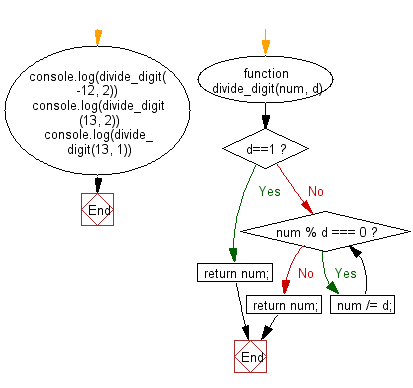# JavaScript: Divide an integer by another integer as long as the result is an integer and return the result

## JavaScript Basic: Exercise-106 with Solution

Write a JavaScript program to divide an integer by another integer as long as the result is an integer and return the result.

Sample Solution:

HTML Code:

``````<!DOCTYPE html>
<html>
<meta charset="utf-8">
<meta name="viewport" content="width=device-width">
<title> Divide an integer by another integer as long as the result is an integer and return the result</title>
<body>

</body>
</html>
```
```

JavaScript Code:

``````function divide_digit(num, d) {
if (d==1)
return num;
else
{
while (num % d === 0) {
num /= d;
}
return num;
}
}
console.log(divide_digit(-12, 2))
console.log(divide_digit(13, 2))
console.log(divide_digit(13, 1))
``````

Sample Output:

```-3
13
13
```

Flowchart:ES6 Version:

``````function divide_digit(num, d) {
if (d==1)
return num;
else
{
while (num % d === 0) {
num /= d;
}
return num;
}
}
console.log(divide_digit(-12, 2))
console.log(divide_digit(13, 2))
console.log(divide_digit(13, 1))
``````

Live Demo:

See the Pen javascript-basic-exercise-106 by w3resource (@w3resource) on CodePen.

What is the difficulty level of this exercise?

Test your Programming skills with w3resource's quiz.

﻿

## JavaScript: Tips of the Day

Converts a string to title case

Example:

```const toTitleCase = str =>
str
.match(/[A-Z]{2,}(?=[A-Z][a-z]+[0-9]*|\b)|[A-Z]?[a-z]+[0-9]*|[A-Z]|[0-9]+/g)
.map(x => x.charAt(0).toUpperCase() + x.slice(1))
.join(' ');
console.log(toTitleCase('some_database_field_name')); // 'Some Database Field Name'
console.log(toTitleCase('Some label that needs to be title-cased')); // 'Some Label That Needs To Be Title Cased'
console.log(toTitleCase('some-package-name')); // 'Some Package Name'
console.log(toTitleCase('some-mixed_string with spaces_underscores-and-hyphens')); // 'Some Mixed String With Spaces Underscores And Hyphens'
```

Output:

```"Some Database Field Name"
"Some Label That Needs To Be Title Cased"
"Some Package Name"
"Some Mixed String With Spaces Underscores And Hyphens"
```